This test lets you check analysis results for a beam with an applied rotation at one of its extremity. You will use either the 2D or 3D model.Reference:

Analytical solution

##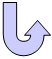Specifications

### Geometry Specifications

 Length: L = 0.5 m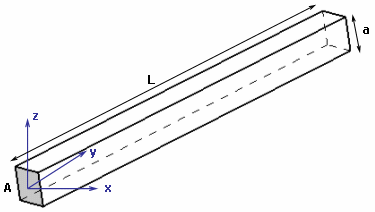Square section: a = 0.02 m

### Analysis Specifications

 Young Modulus (material): E = 200 GPa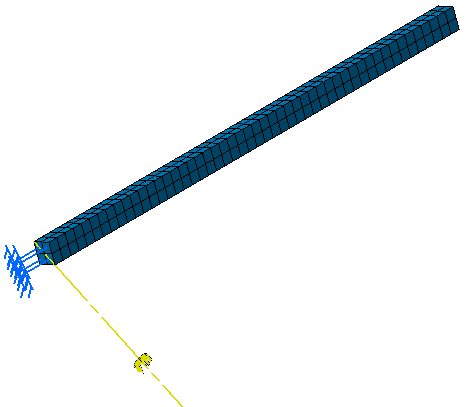Poisson's Ratio (material): ν = 0 Density: ρ = 7800 kg/m-3 Mesh Specifications: 3D model: 2 x 2 x 50 linear hexahedron elements (HE8) 2D model: 4 x 8 linear quadrangle elements (QD4) Restraints: Clamp on face A: Tx = Ty = Tz = Rx = Ry = Rz = 0 Loads (rotation force): Angular Velocity: 3000 rad/s Angular Acceleration: a=0 rad/s2 The direction of the rotation axis through the center of the clamped section A is (1,0,-1).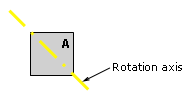##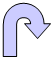Results

• Analytical solution

 In the beam axis: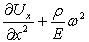where: Ux(0)=0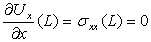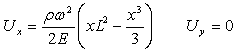The displacements of all the points of the beam in the global axis are given by: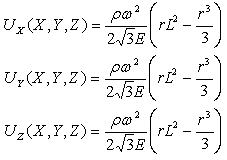where: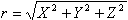For r=500 mm, the result of the analytic solution is: Ux=Uy=Uz=8.44374 mm
• The table below presents the analysis results:

 Displacement Analytical solution  [mm] Values Linear Hexahedron (HE8) Linear Quadrangle (QD4)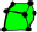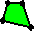Computed Results  [mm] Normalized Results Computed Results  [mm] Normalized Results Ux 8.44374 8.44374 1 8.44372 0.999998 Uy 8.44374 8.44377 1.000004 8.44377 1.000004 Uz 8.44374 8.44374 1 8.44376 1.000002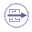To Perform the Test:

The rotationloadingHE8.CATAnalysis document presents a complete analysis, computed with a mesh formed of linear hexahedron elements (HE8).

The rotationloadingQD4.CATAnalysis document presents a complete analysis, computed with a mesh formed of linear quadrangle elements (QD4).

To compute the case with linear quadrangle elements (QD4) and linear hexahedron elements (HE8), proceed as follow:

1. Open one of the CATAnalysis documents.

2. Compute the case and activate the Deformed mesh.1 and Translational displacement vector.1 images called Deformed mesh.